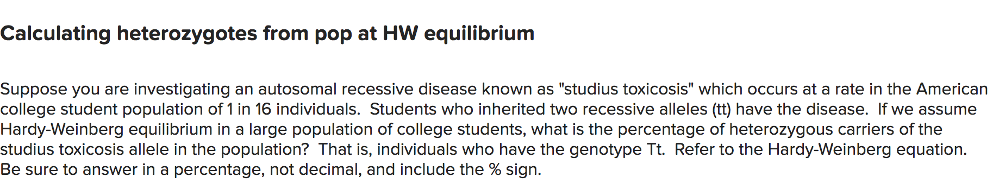# Problem: Suppose you are investigating an autosomal recessive disease known as "studious toxicosis" which occurs at a rate in the American college student population of 1 in 16 individuals. Students who inherited two recessive alleles (tt) have the disease. If we assume Hardy-Weinberg equilibrium in a large population of college students, what is the percentage of heterozygous carriers of the studious toxicosis allele in the population?

###### FREE Expert Solution

If a trait is at Hardy-Weinberg equilibrium, it should follow the equation p2 + 2pq + q2 = 1, where p2 is the gentoypic frequency for the homozygous dominant, 2pq is for heterozygous frequency, and q2 is for homozygous recessive frequency.###### Problem Details

Suppose you are investigating an autosomal recessive disease known as "studious toxicosis" which occurs at a rate in the American college student population of 1 in 16 individuals. Students who inherited two recessive alleles (tt) have the disease. If we assume Hardy-Weinberg equilibrium in a large population of college students, what is the percentage of heterozygous carriers of the studious toxicosis allele in the population?